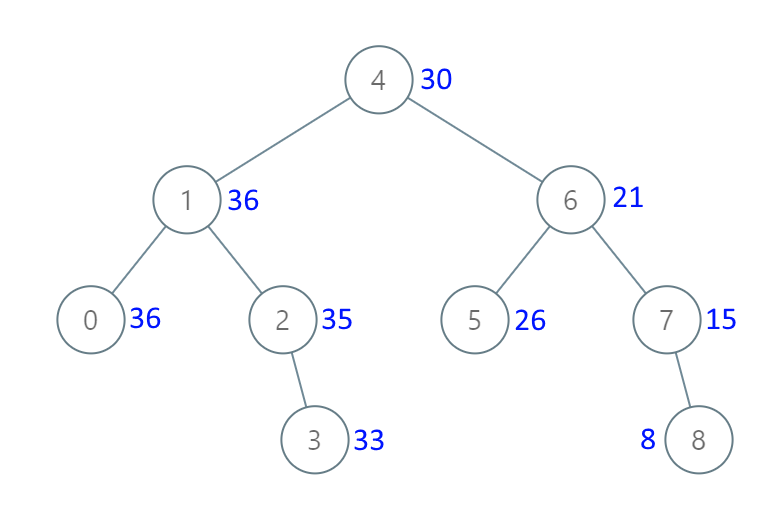## Algorithm

Problem Name: 1038. Binary Search Tree to Greater Sum Tree

Given the `root` of a Binary Search Tree (BST), convert it to a Greater Tree such that every key of the original BST is changed to the original key plus the sum of all keys greater than the original key in BST.

As a reminder, a binary search tree is a tree that satisfies these constraints:

• The left subtree of a node contains only nodes with keys less than the node's key.
• The right subtree of a node contains only nodes with keys greater than the node's key.
• Both the left and right subtrees must also be binary search trees.

Example 1:```Input: root = [4,1,6,0,2,5,7,null,null,null,3,null,null,null,8]
Output: [30,36,21,36,35,26,15,null,null,null,33,null,null,null,8]
```

Example 2:

```Input: root = [0,null,1]
Output: [1,null,1]
```

Constraints:

• The number of nodes in the tree is in the range `[1, 100]`.
• `0 <= Node.val <= 100`
• All the values in the tree are unique.

## Code Examples

### #1 Code Example with Java Programming

```Code - Java Programming```

``````
class Solution {
public TreeNode bstToGst(TreeNode root) {
int sum = 0;
TreeNode node = root;
Stack stack = new Stack<>();
while (!stack.isEmpty() || node != null) {
while (node != null) {
node = node.right;
}
node = stack.pop();
sum += node.val;
node.val = sum;
node = node.left;
}
return root;
}
}
``````
Copy The Code &

Input

cmd
root = [4,1,6,0,2,5,7,null,null,null,3,null,null,null,8]

Output

cmd
[30,36,21,36,35,26,15,null,null,null,33,null,null,null,8]

### #2 Code Example with Javascript Programming

```Code - Javascript Programming```

``````
const bstToGst = function(root) {
const arr = []
dfs(root, arr)
let v = 0
for(let i = arr.length - 1; i >= 0; i--) {
arr[i].val = arr[i].val + v
v = arr[i].val
}
return root
};

function dfs(node, arr) {
if(node == null) return
dfs(node.left, arr)
arr.push(node)
dfs(node.right, arr)
}
``````
Copy The Code &

Input

cmd
root = [4,1,6,0,2,5,7,null,null,null,3,null,null,null,8]

Output

cmd
[30,36,21,36,35,26,15,null,null,null,33,null,null,null,8]

### #3 Code Example with Python Programming

```Code - Python Programming```

``````
class Solution:
val = 0
def bstToGst(self, root):
if root.right: self.bstToGst(root.right)
root.val = self.val = self.val + root.val
if root.left: self.bstToGst(root.left)
return root
``````
Copy The Code &

Input

cmd
root = [0,null,1]

Output

cmd
[1,null,1]

### #4 Code Example with C# Programming

```Code - C# Programming```

``````
namespace LeetCode
{
public class _1038_BinarySearchTreeToGreaterSumTree
{
private int sum = 0;

public TreeNode BstToGst(TreeNode root)
{
if (root != null)
{
BstToGst(root.right);
sum += root.val;
root.val = sum;
BstToGst(root.left);
}

return root;
}
}
}
``````
Copy The Code &

Input

cmd
root = [0,null,1]

Output

cmd
[1,null,1]